# pyspark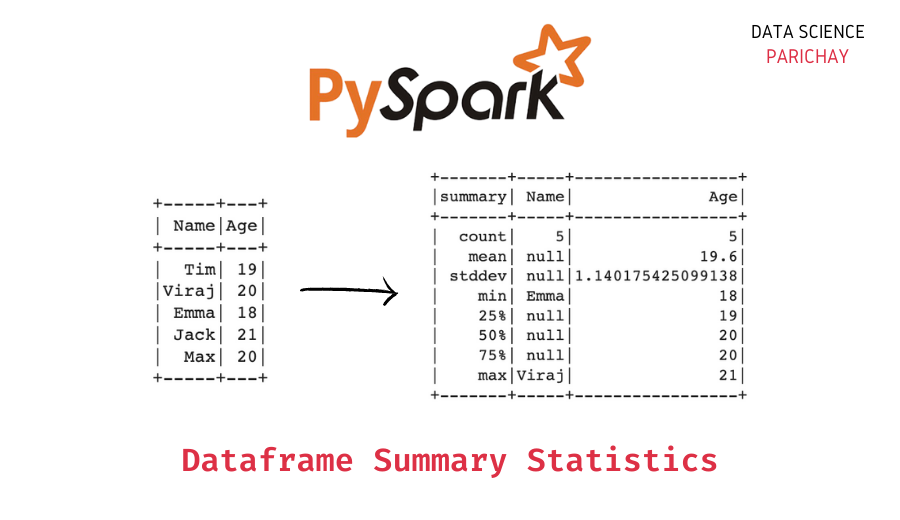## Get Pyspark Dataframe Summary Statistics

In this tutorial, we will look at how to get the summary statistics for a Pyspark dataframe with the help of some examples. How to get the summary statistics of a Pyspark dataframe? You can use the Pyspark dataframe summary() function to get the summary statistics for a dataframe in Pyspark. The following is the …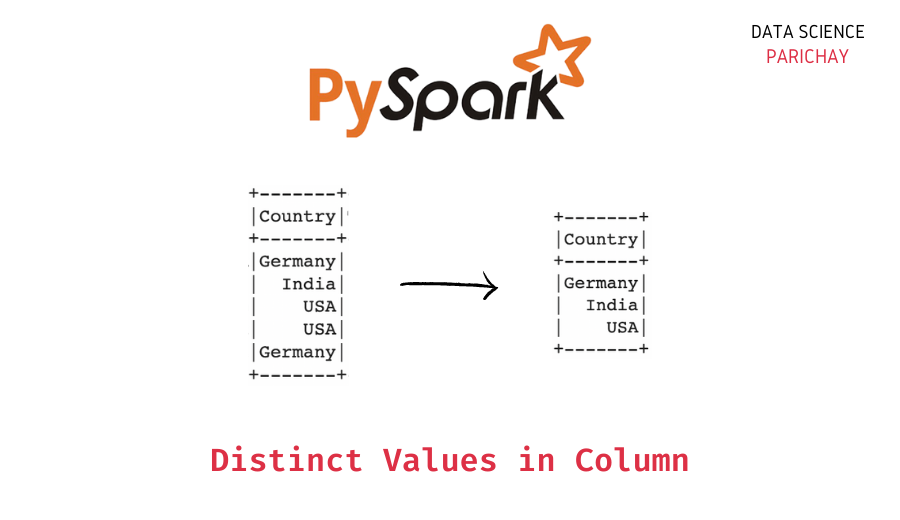## Pyspark – Get Distinct Values in a Column

In this tutorial, we will look at how to get the distinct values in a Pyspark column with the help of some examples. How to get distinct values in a Pyspark column? You can use the Pyspark distinct() function to get the distinct values in a Pyspark column. The following is the syntax – Here, …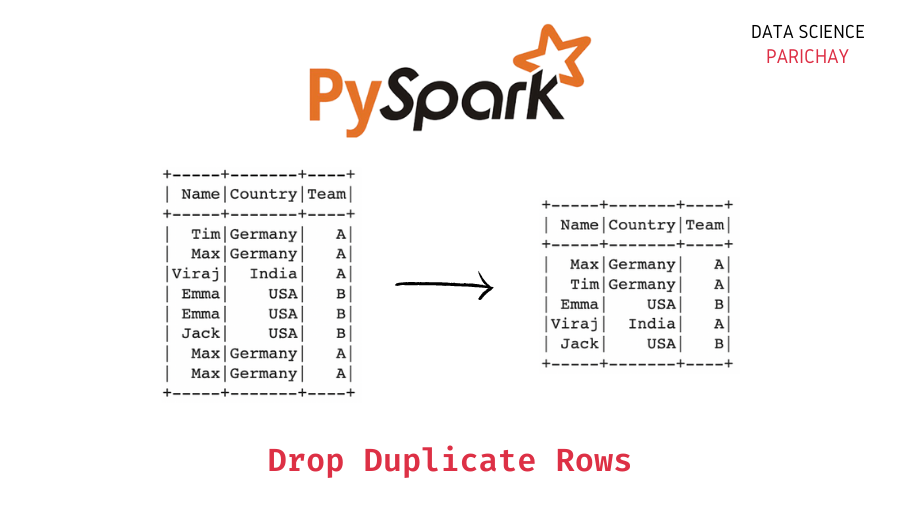## Drop Duplicate Rows from Pyspark Dataframe

In this tutorial, we will look at how to drop duplicate rows from a Pyspark dataframe with the help of some examples. How to drop duplicate rows in Pyspark? You can use the Pyspark dropDuplicates() function to drop duplicate rows from a Pyspark dataframe. The following is the syntax – Apply the function on the …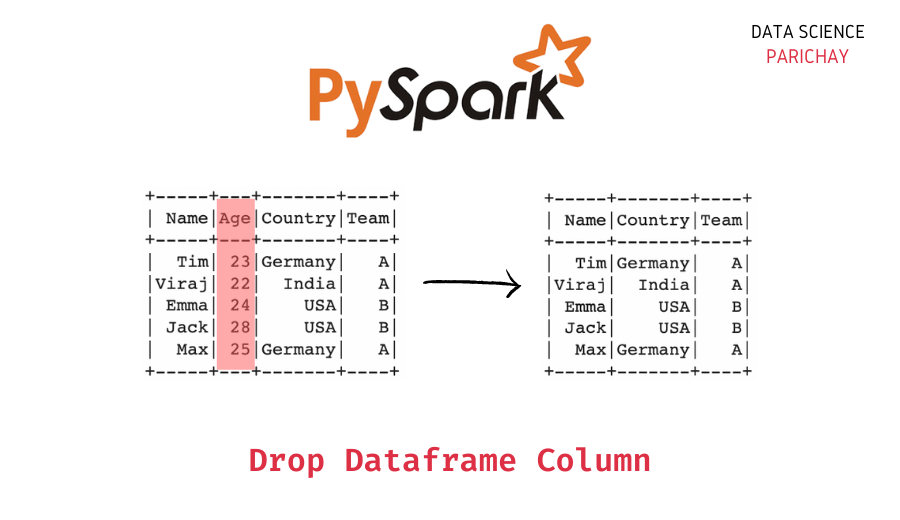## Drop One or More Columns From Pyspark DataFrame

In this tutorial, we will look at how to drop one or more columns from a Pyspark dataframe with the help of some examples. How to drop Pyspark dataframe columns? You can use the Pyspark drop() function to drop one or more columns from a Pyspark dataframe. Pass the column (or columns) you want to …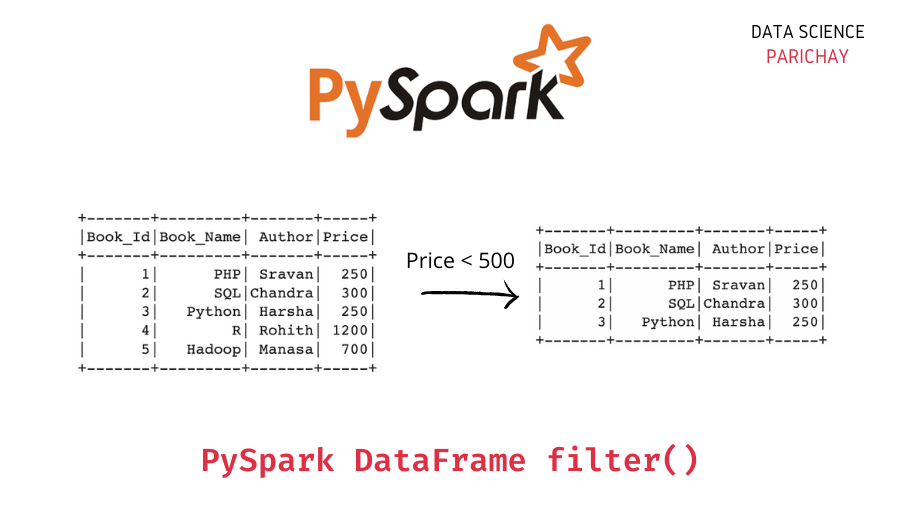## Filter Pyspark Dataframe with filter()

In this tutorial, we will look at how to filter data in a Pyspark dataframe with the help of some examples. How to filter data in a Pyspark dataframe? You can use the Pyspark dataframe filter() function to filter the data in the dataframe based on your desired criteria. The following is the syntax – …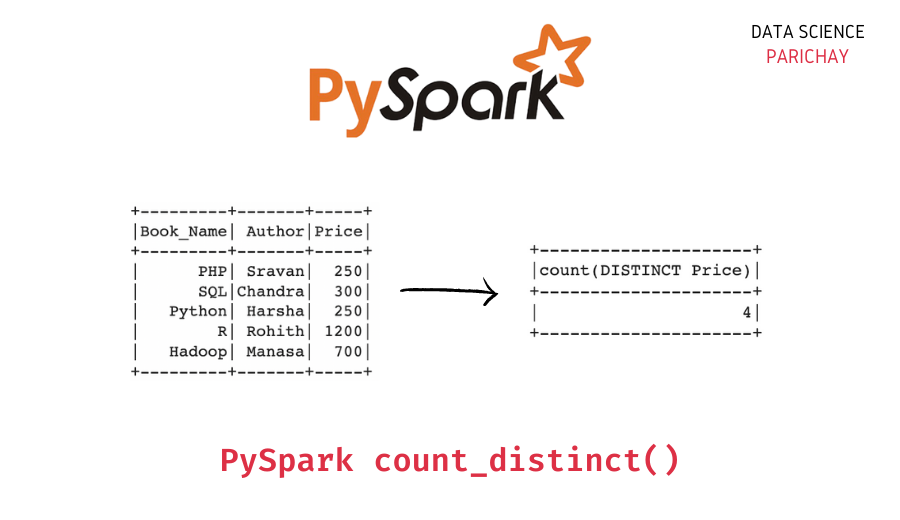## Pyspark – Count Distinct Values in a Column

In this tutorial, we will look at how to get a count of the distinct values in a column of a Pyspark dataframe with the help of examples. How to count unique values in a Pyspark dataframe column? You can use the Pyspark count_distinct() function to get a count of the distinct values in a …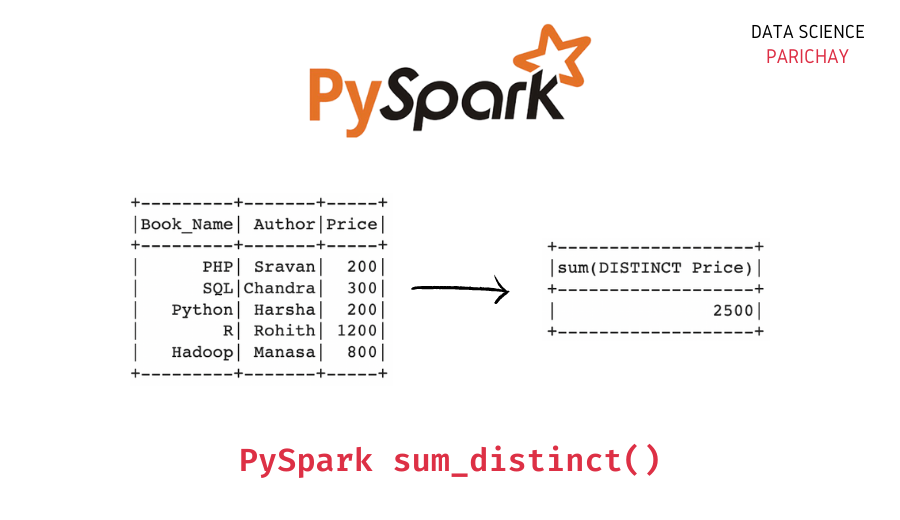## Pyspark – Sum of Distinct Values in a Column

In this tutorial, we will look at how to get the sum of the distinct values in a column of a Pyspark dataframe with the help of examples. How to sum unique values in a Pyspark dataframe column? You can use the Pyspark sum_distinct() function to get the sum of all the distinct values in …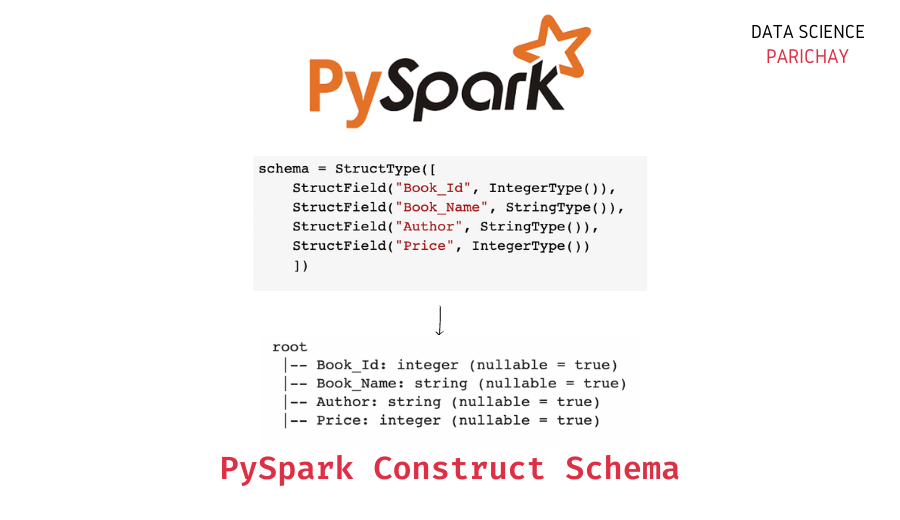## Pyspark DataFrame Schema with StructType() and StructField()

In this tutorial, we will look at how to construct schema for a Pyspark dataframe with the help of Structype() and StructField() in Pyspark. Pyspark Dataframe Schema The schema for a dataframe describes the type of data present in the different columns of the dataframe. Let’s look at an example. Output: Here, we created a …

Scroll to Top